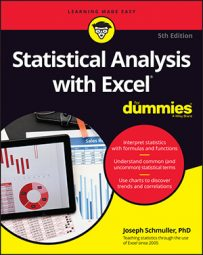##### Statistical Analysis with Excel For DummiesIn addition to Excel’s built-in array formulas, you can create your own. To help things along, you can incorporate named arrays. The image below shows two named arrays, X and Y, in columns C and D, respectively. X refers to C2 through C5 (not C1 through C5), and Y refers to D2 through D5 (not D1 through D5). XY is the column header for column F. Each cell in column F will store the product of the corresponding cell in column C and the corresponding cell in column D.

An easy way to enter the products, of course, is to set F2 equal to C2*E2 and then autofill the remaining applicable cells in column F.

Just to illustrate array formulas, though, follow these steps to work on the data in the worksheet.

1. Select the array that will hold the answers to the array formula. That would be F2 through F5 — or F2:F5, in Excel-speak.
2. Into the selected array, type the formula. The formula here is `=X * Y`
3. Press Ctrl+Shift+Enter (not Enter). On the Mac, that’s Ctrl+Shift+Return or Command+Shift+Return. The answers appear in F2 through F5. Note the formula `{=X*Y}` in the Formula bar. The curly brackets indicate an array formula.
When you name a range of cells, make sure that the named range does not include the cell with the name in it. If it does, an array formula like `{=X * Y}` tries to multiply the letter X by the letter Y to produce the first value, which is impossible and results in the exceptionally ugly `#VALUE!` error.

The weird Mac behavior with regard to the `FREQUENCY` array formula does not occur in a homemade array formula — it’s not necessary to click the Formula bar before pressing the keystroke combination.

Command+clicking the Enter button (the check mark next to the Formula bar) works in this context, too.